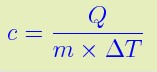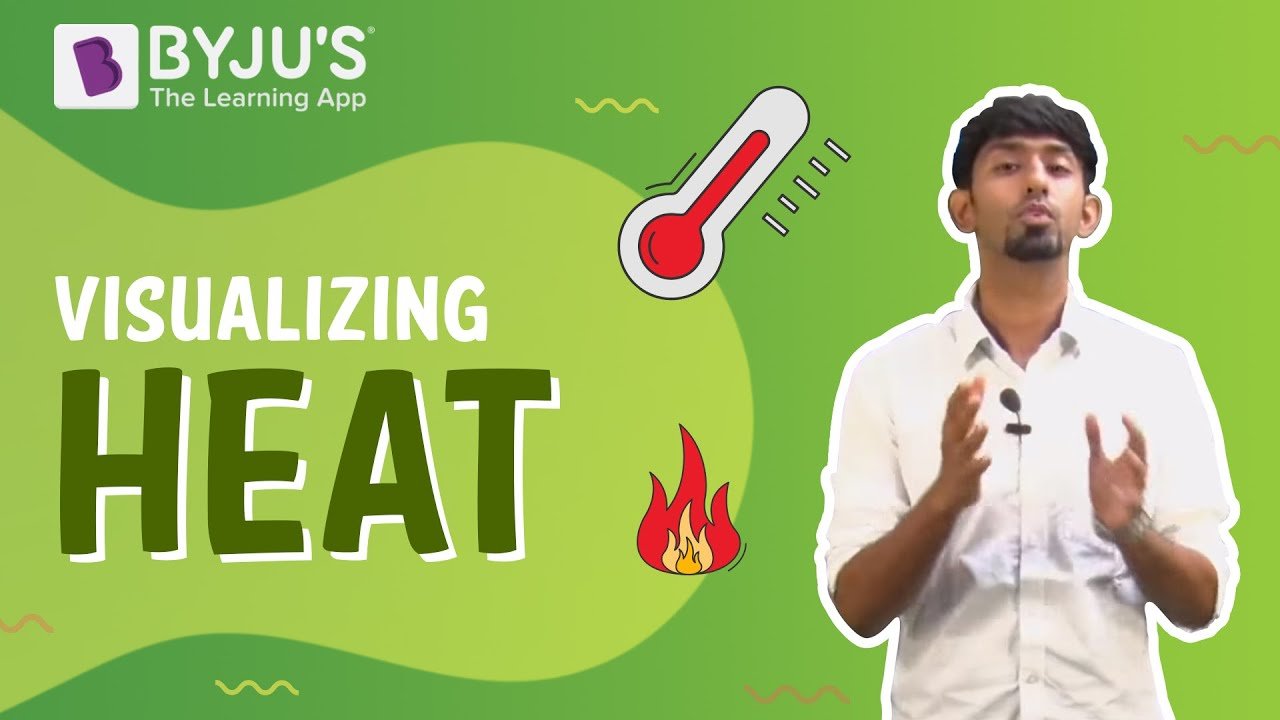# Unit of Heat

We are all familiar with the term heat, and we experience the effect of heat almost every day in our lives. As we are so familiar with the topic if we look at the science behind it, heat is the form of energy that changes the temperature in any substance. Heat involves the transfer of energy from an object or an energy source to another medium or an object. Heat is represented by the symbol Q and the heat formula is given as;Where,
M = mass of the body,
C = specific heat,
Δ T = temperature difference.

While we have learned about what heat is in quick succession, it is also important to learn about how it is measured and the unit of heat. Let’s discuss them below.

## SI Unit of Heat

Generally, in the SI system, all forms of energy are measured in terms of joules. Notably, heat is a form of energy, and therefore the SI unit of heat is also joules (J) which are defined as the amount of energy needed to raise the temperature of a given mass by one degree. Usually, 4.184 joules of heat energy is necessary to increase the temperature of a unit weight (say 1 g) of water from 0 degrees to 1 degree Celsius.

### Other Heat Units

In the CGS system, heat is expressed in the unit of calories which is further said to be the heat energy needed to increase the temperature of 1 gm of clean water by one degree Celsius. Sometimes kilocalorie (kcal) is also referred to as a unit of heat where 1 kcal = 1000 cal.

Additionally, the British thermal unit (BTU), which is part of the imperial system, is also used to measure or calculate heat.

 Units of Heat Calorie 1 cal 4184 J Joules 1 J 0.000239006 kcal / 0.000947817Btu BTU 1 Btu 1055.06 J

Related articles:

## What is heat? Why do we experience it? How does it travel?Stay tuned to BYJU’S and Fall in Love with Learning!

Test your Knowledge on unit of heat

1 joule= erge

2. Shankara Vulli

Yes

3. i asked define the units of heats

1. sushmitha

Following are the three units of heat:
Joules
Calorie
BTU (British Thermal Unit)